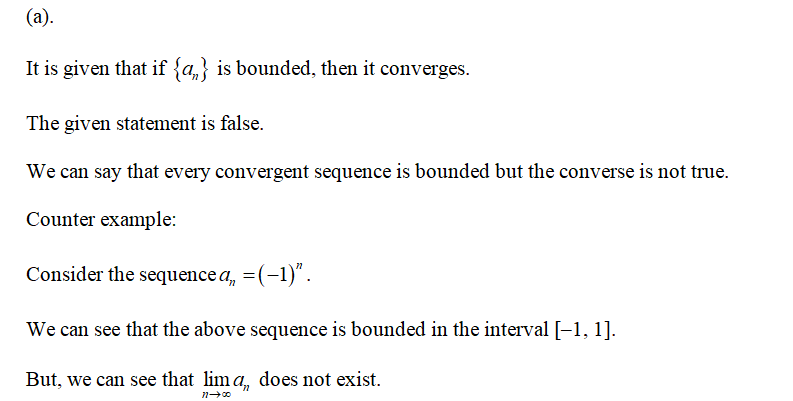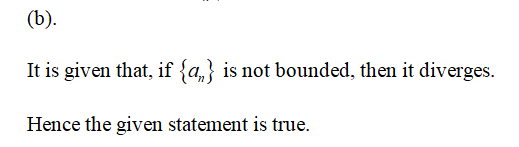# Theorem 5 says that every convergent sequence is bounded. Determine if the following statements are true or false, and if false, give a counterexample: (a) If {an} is bounded, then it converges. (b) If {an} is not bounded, then it diverges. (c) If {an} diverges, then it is not bounded.

Question
1 views

Theorem 5 says that every convergent sequence is bounded. Determine if the following statements are
true or false, and if false, give a counterexample:
(a) If {an} is bounded, then it converges.
(b) If {an} is not bounded, then it diverges.
(c) If {an} diverges, then it is not bounded.

check_circle

Step 1Step 2...

### Want to see the full answer?

See Solution

#### Want to see this answer and more?

Solutions are written by subject experts who are available 24/7. Questions are typically answered within 1 hour.*

See Solution
*Response times may vary by subject and question.
Tagged in